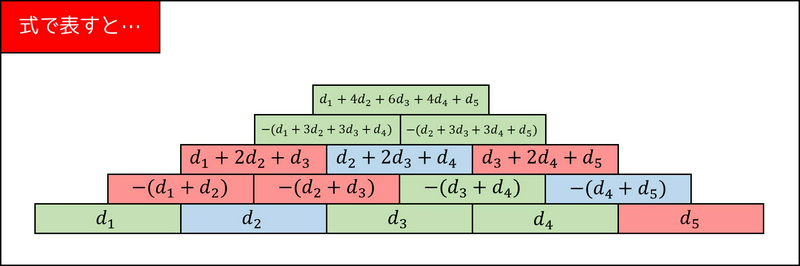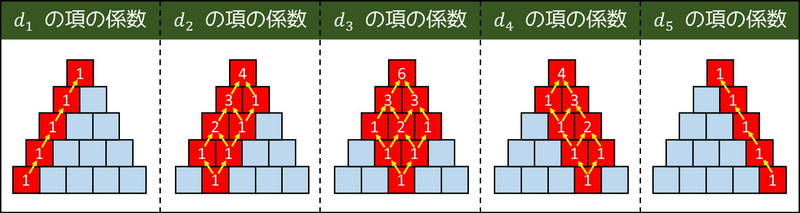## C - Tricolor Pyramid 解説 by evima

Let us call the colors $$0, 1, 2$$. Then, on top of two blocks of colors $$a$$ and $$b$$, a block of color $$-(p_1 + p_2) \bmod 3$$ will be placed.

Thus, the answer is $$\left(-1\right)^{N-1} \times \sum_{i=0}^{N} (c_{i-1} \times {}_{N-1}C_{i-1})$$, modulo $$3$$. (See figures below.)To compute a binomial coefficient $${}_{n}C_{r}$$ modulo $$3$$, compute the values $$f(n!), f(r!), f((n - r)!), g(n!), g(r!), g((n - r)!)$$, where $$f(m)$$ is the number of times $$m$$ can be divided by $$3$$, and $$g(m)$$ is the result, modulo $$3$$, of dividing $$m$$ by $$3$$ until it is no longer divisible by $$3$$. For a given integer $$m$$, we can compute $$f(m!)$$ and $$g(m!)$$ in $$O(\log m)$$ time.

Sample Implementation in C++: https://atcoder.jp/contests/arc117/submissions/21732692